> > Introduction to Decimal

# Introduction to Decimal

Decimals have always been a problematic subject for the students. Many simply fail to understand the usability and its need for our study. But like everything that you are taught, it has a need and importance of their own as well. So, before we talk about decimals, you need to understand why was there ever a need for it? Let’s begin.

### Suggested VideosIntroduction to decimal numbersDecimal numbers on number lineComparing Decimal Numbers## Analysis

Let’s start with an example. Order the numbers below from least to greatest: 58, 57 and 57$$\frac{49}{100}$$. As you already know that, 57$$\frac{49}{100}$$ is a mixed number. It comprises a fraction and a whole number. Now, let us use the place value that will help you compare these numbers. So,

57 = (5 × 10) + (7 × 1)
57$$\frac{49}{100}$$ = (5 × 10) + ( 7 × 1) + (4 × $$\frac{1}{10}$$ ) + (9 × $$\frac{1}{100}$$)
58= (5 × 10) + (8 × 1)

Answer: Ordering these numbers from least to greatest, we get: 57, 57$$\frac{49}{100}$$ and 58. But that’s like a lot of writing! So, what do we do now? In order to avoid this situation, we can use decimals to write 57$$\frac{49}{100}$$.

## What is a Decimal?A decimal is any number from the base-ten number system. In this unit, we’ll be specifically focussing on the numbers that have one or more digits to the right of the point. The decimal point helps in separating the ones place from the tenths place in the number.

In money, the point is used to separate dollars from cents. When we move to the right of the point, every number place is divided by 10. So, now we’ll express the number 57 $$\frac{49}{100}$$ in the decimal form and the expanded form. The number is 57 $$\frac{49}{100}$$.

First, let’s write the mixed number in the expanded form = (5 x 10) + ( 7 x 1) + (4 × $$\frac{1}{10}$$ ) + (9 × $$\frac{1}{100}$$). Hence, the decimal form of this number is  ‘57.49’. It is very clear that writing the number in a decimal form is significantly easier. Now, we’ll write this number in the place value chart to give you a better understanding of how the decimals work.

## Value and Decimals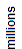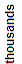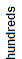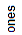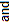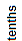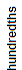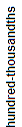5 7 . 4 9

When you move right in this place value chart, every number is divided by 10. For instance, thousands are divided by 10 to give you hundreds. This also stands true for the digits that are on the right of the decimal point.  For instance, tenths, when divided by 10, give you hundredths. However, keep in mind that when you read the decimals, the decimal point must be read as “and.”

So, if the decimal number is 57.49, it is read as “fifty-seven and forty-nine hundredths.” However, in the day to day life, we read the decimal point as “point” and not “and”. So, the  decimal number 57.49 is read as “fifty-seven point four nine.” However, this usage isn’t mathematically correct. Let’s understand this with an example

Write each phrase as a fraction and as a decimal.

 Phrase Fraction Number six tenths $$\frac{6}{10}$$ 0.6 five hundredths $$\frac{5}{100}$$ 0.05 thirty-two hundredths $$\frac{32}{100}$$ 0.32 two hundred sixty-seven thousandths $$\frac{267}{1000}$$ 0.267

## Why are Decimals Used?

The decimals are used in cases that require more precision than can be provided by the whole numbers. One of the best examples of this is money. When we say three and one-fourth dollars, it is an amount that lies between 3 dollars and 4 dollars. So, this amount can be expressed as \$3.25.

The decimal number has a fractional part and a whole number part. Whole number part is the digits on the left of the point. On the other hand, the fractional part is the digits that are on the right of the point.  This point is used to separate these two parts. Let’s understand this better with the help of examples.

 Number Whole-Number Part Fractional Part 3.25 3 25 4.172 4 172 25.03 25 03 0.168 0 168 132.7 132 7

As shown above, you can also express these decimal numbers on the place value chart. We will, however, leave that for you to do. Please note, that 0.168 and .168 mean the same and have the same value. However, it is better to use zero before the decimal point.

This will provide us with clarity on the fact that the number provided is lesser than one. So, from now on, whenever you are writing a decimal which has value lesser than one, you have to include a zero in the ones place. Let’s provide more clarity on this with some more examples of the decimals.

Example 2: Write each phrase as a number.

 Phrase Number fifty-six hundredths 0.56 nine-tenths 0.9 thirteen and four hundredths 13.04 twenty-five and eighty-one hundredths 25.81 nineteen and seventy-eight thousandths 19.078

Example 3: Write each number using words.

 Number Phrase 0.005 five thousandths 100.6 one hundred and six tenths 2.28 two and twenty-eight hundredths 71.062 seventy-one and sixty-two thousandths 3.0589 three and five hundred eighty-nine ten-thousandths

## How Long can a Decimal be?

A figure can have any number of decimal places to the right of the point. Now, we’ll explain this with the help of an example of the numerical value of Pi. We have shortened it to 50 decimal digits. Let’s take a look.

Pi = 3.14159 26535 89793 23846 26433 83279 50288 41971 69399 37510

## Decimal Digits

If the number is 1.0897, then the digits, 0, 8, 9 and 7 are the Decimal Digits.  So, the digits that are on the right of the point are called the Decimal Digits.

## Solved Examples for You

Question 1:Which of the following is equal to 5/100?

1. 0.5
2. 5.0
3. 0.05
4. 0.005

Question 2: What is a decimal?

Answer: Decimal refers to a fraction that we write in a special form. For instance, in place of writing ½ you can simply express it in decimal form as 0.5. Here the zero is in the place of ones and five is in the tenths place.

Question 3: What is the place value of the decimal number?

Answer:The number in decimal point has different values than the values of the left of the decimal. For instance, the decimal value of 0.6 the six is in the tenths place. In the same way, in 5.124 on is in the tenths place, two is in the hundreds place, and four is in the thousands place.

Question 4: What is an expanded form?

Answer: It refers to a way of writing numbers to see the math values of individual digits. Moreover, when we separate numbers into individual place values and decimal places then they can also form mathematical expression. Such as, the expanded form of 1245 is 1000 + 200 + 40 + 5 = 1245.

Question 5: Can the percentage be written as a decimal?

Answer: Yes, we can write a percentage in decimal because percentage represents values in hundredths part. For example, 36% means that 36 parts of 100 which we can write in decimal as 0.36.

Share with friends

## Customize your course in 30 seconds

##### Which class are you in?
5th
6th
7th
8th
9th
10th
11th
12th
Get ready for all-new Live Classes!
Now learn Live with India's best teachers. Join courses with the best schedule and enjoy fun and interactive classes.Ashhar Firdausi
IIT Roorkee
BiologyDr. Nazma Shaik
VTU
ChemistryGaurav Tiwari
APJAKTU
Physics
Get Started

## Browse

##### Decimals
• Introduction to Decimal0 Followers

Most reacted comment
1 Comment authorsRecent comment authors
Subscribe
Notify ofGuest
Andre

What is the decimal rule for final configuration – Is 5.3 = 5

## Browse

##### Decimals
• Introduction to Decimal

## Question Mark?

Have a doubt at 3 am? Our experts are available 24x7. Connect with a tutor instantly and get your concepts cleared in less than 3 steps.